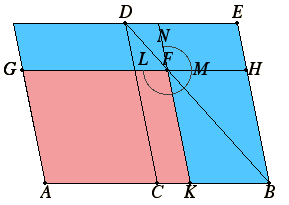# Proposition 27

Of all the parallelograms applied to the same straight line falling short by parallelogrammic figures similar and similarly situated to that described on the half of the straight line, that parallelogram is greatest which is applied to the half of the straight line and is similar to the difference.

Let AB be a straight line and let it be bisected at C. Let there be applied to the straight line AB the parallelogram AD falling short by the parallelogrammic figure DB described on the half of AB, that is, CB.

I say that, of all the parallelograms applied to AB falling short by parallelogrammic figures similar and similarly situated to DB, AD is greatest.Let there be applied to the straight line AB the parallelogram AF falling short by the parallelogrammic figure FB similar and similarly situated to DB.

I say that AD is greater than AF.

VI.26

Since the parallelogram DB is similar to the parallelogram FB, therefore they are about the same diameter.

Draw their diameter DB, and describe the figure.

I.43

Then, since CF equals FE, and FB is common, therefore the whole CH equals the whole KE.

I.36

But CH equals CG, since AC also equals CB.

Therefore CG also equals KE.

Add CF to each. Therefore the whole AF equals the gnomon LMN, so that the parallelogram DB, that is, AD, is greater than the parallelogram AF.

Therefore, of all the parallelograms applied to the same straight line falling short by parallelogrammic figures similar and similarly situated to that described on the half of the straight line, that parallelogram is greatest which is applied to the half of the straight line and is similar to the difference.

Q.E.D.

## Guide

This proposition clarifies the limitations of the next one, VI.28. In VI.28 a construction is made to apply a parallelogram equal to a given rectilinear figure to a line falling short by a parallelogrammic figure. This proposition implies that that construction cannot be made if the given rectilinear figure is too large.

When that proposition is applied, the part which falls short is usually a square, not just any parallelogram, and this and the next proposition are much more easily understood in that case. In that case, the next proposition applies a rectangle equal to a given area to a line but falling short by a square. And this proposition implies that can only be done if the given area is at least the square on half the line, since that square is the greatest rectangle that can be so applied.